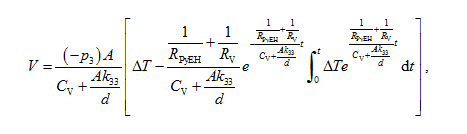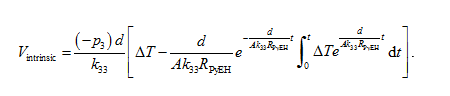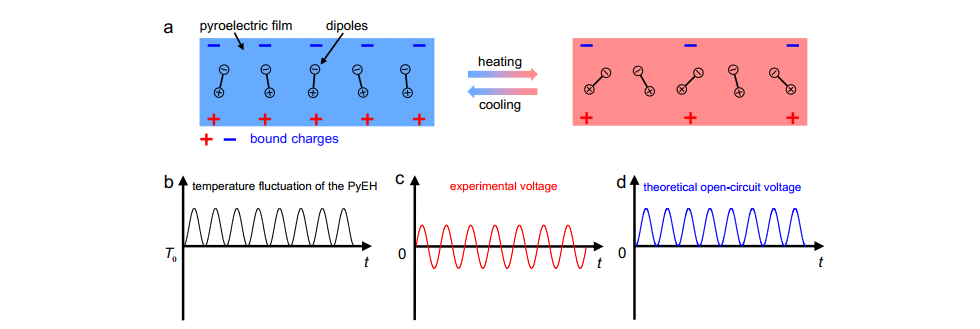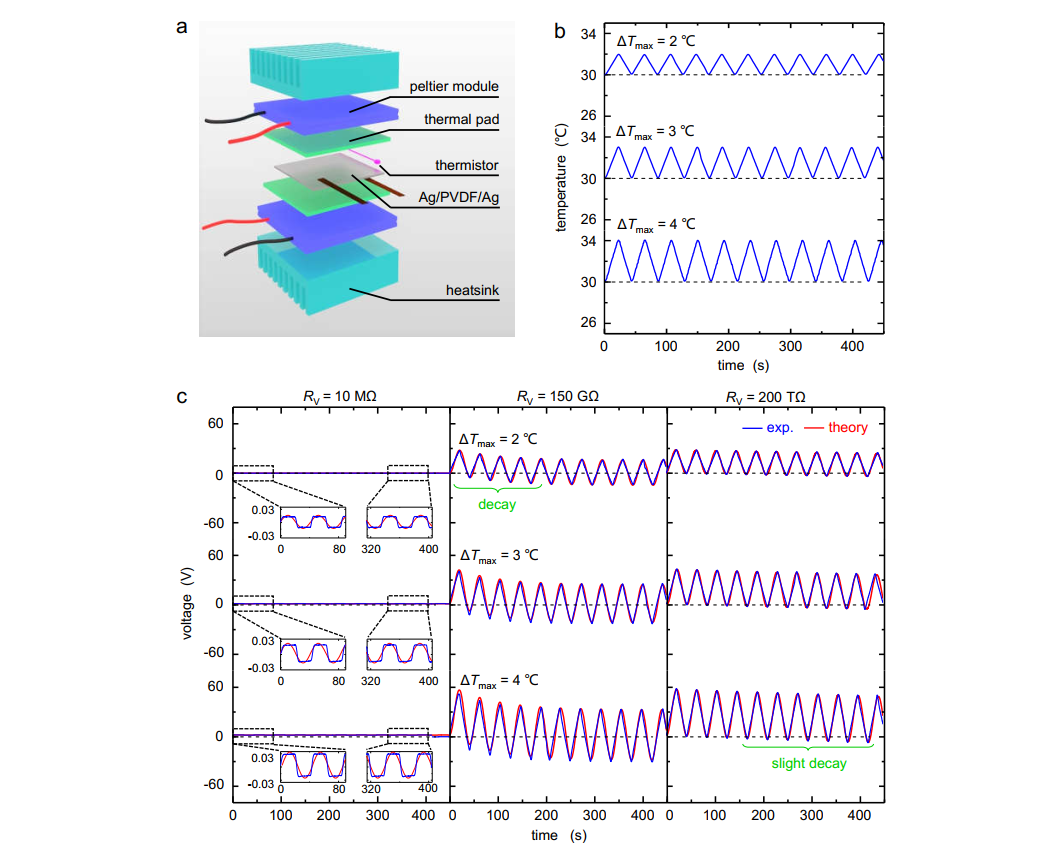## On the evaluation of the performance of pyroelectric energy harvesters

Date：2021-09-09Source：

Wireless, low-power advanced electronic devices like the wearable sensors have attracted extensive interest due to their wide range of potential applications in recent years. The systems consisting of advanced energy harvesters and new energy storage technologies, such as supercapacitors, are investigated for the energy supply enabling continued operation of these devices, whereas conventional batteries are generally too bulky and environmentally unfriendly to power miniaturized and widely utilized components.

The pyroelectric energy harvester (PyEH), which can convert the temperature fluctuation into electrical energy, is one of the most popular classes of devices in this framework. As shown in Fig. 1a, the electrical potential difference is yielded between the top and bottom surfaces, when a pyroelectric film with polarization direction along the thickness direction is subjected to heating or cooling. The output voltage under the temperature change of 1 (V/℃) is a critical parameter to determine the performance of the PyEH. Some results also named it as the open-circuit voltage. However, by the pyroelectric theory of the open circuit, the open-circuit voltage should be proportional to the temperature fluctuation (Fig. 1b and 1d), which significantly conflicts with the experimental findings (Fig. 1c). To address this critically important problem, Prof. Yewang Su et al. both investigated the theory model and carried out experimental measurements.

A PyEH was fabricated based on the PVDF film, and applied a temperature fluctuation, as shown in Fig. 2a and 2b. Three voltmeters with different inner resistance value RV = 10 MΩ, 150 GΩ, and 200 TΩ are used to measure the voltage of the same PyEH, respectively. As shown in Fig. 2c, three different modes of the voltage response are obtained and the peak values of the first period are 0.0247 V, 49.9 V, and 56.7 V for the voltmeter with resistances RV = 10 MΩ, 150 GΩ, and 200 TΩ, respectively, when the temperature fluctuation ΔTmax = 4 is the same for all cases. The peak value of the voltage output significantly increases with the resistance of the voltmeter, and the difference can be as large as 2296 times. The measured values so depend on the instrument used, which is contrary to the established assumption of set-up independent experimental results.

To understand this issue, the relation between the temperature fluctuation and the pyroelectric voltage considering the influence of the resistance and the capacitance of both the voltmeter and the PyEH was conducted:where p3, d and A are the pyroelectric coefficient, thickness, and the surface area of the PyEH, respectively. RPyEH, k33, RV, and CV are the resistance and the permittivity of the PyEH, the resistance and the capacitance of the voltmeter, respectively. The theoretical results agree very well with the experimental findings, including both peaks and shapes of the voltage–time curves. The conclusion that the pyroelectric charge would “leak” through the resistance of the voltmeter was obtained. However, even a 200-TΩ-resistance voltmeter was used, the decay of the voltage can be still observed. This is due to that the PyEH itself has a finite resistance, which means the open-circuit condition cannot be obtained in many cases. Therefore, the intrinsic voltage was proposed to replace the open-circuit voltage as the standard to evaluate the performance of the PyEH:In the meantime, two other important conclusions were obained: 1) The output voltage is sensitive to the temperature rate rather than the peak of temperature fluctuation when a 10-MΩ-resistance is used. This means the results of output voltage per degree Celsius cannot reflect the device performance in many previous works in which the voltmeter with a small resistance was used. 2) The output voltage is very sensitive to the capacitance of the voltmeter when a 200-TΩ resistance voltmeter is used, while this parameter was not given in many previous works in which the voltmeter with a large resistance was used.

The above work was published in nano energy (https://doi.org/10.1016/j.nanoen.2021.106045). The first author and the corresponding author are Ph.D candidate Qinlan Li and Prof. Yewang Su, respectively. This work was supported by the National Natural Science Foundation of China, Key Research Program of Frontier Sciences of the Chinese Academy of Sciences, CAS Interdisciplinary Innovation Team, and Strategic Priority Research Program of the Chinese Academy of Sciences.Fig. 1. The confliction between the experimental and theoretical voltage-time curves. (a) Schematic illustration of the basic mechanism of operation of pyroelectric materials. Schematic illustrations of the curves of (b) the temperature fluctuation applied to the PyEH, output voltage versus time from (c) experiments and (d) the pyroelectric theory for the open circuit.Fig. 2. Experimental results and theoretical prediction of the output voltage of the PyEH. (a) Schematic illustration of the temperature controller for the performance characterization of the PyEH. (b) Temperature fluctuation applied to the PyEH. (c) Voltage curves of the same PyEH measured by three voltmeters with inner resistances of 10 MΩ (left), 150 GΩ (middle), and 200 TΩ (right), respectively.